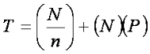# Powers Group

## Determining the Optimal Mixture Size for NMR-Based Screens

In order to increase the efficiency of NMR-based protein-ligand binding screens, small molecules are often screened as mixtures. Generally, mixture sizes have been arbitrarily chosen to contain 5-10 compounds. An analysis of the efficiency of mixtures and a corresponding determination of the optimum mixture size (OMS) that minimizes the amount of material and instrumentation time required for an NMR screen has been lacking. A model for calculating OMS based on the application of the hypergeometric distribution function to determine the probability of a "hit" for various mixture sizes and hit rates has been determined.

### Hypergeometric distribution functionWhere P is the probability of a mixture containing at least one hit, x is the number of hits present in a mixture, n is the number of compounds in the mixture, M is the number of hits present in the library, and N is the total population of the library.

### Impact of DeconvolutionWhere T is the total number NMR experiments collected to identify binders in a library.

The OMS is strongly hit-rate dependent and drops dramatically as the hit-rate approaches typical values (~5%) observed for directed or focused chemical libraries. Since the efficiency of a screen (minimal number of NMR experiments) is always greater in the absence of deconvolution, it is always better to assay a directed library using small mixture sizes (3-4) that does not require deconvolution.

### Picture Gallery

• From: Journal of Biomolecular NMR (2005)